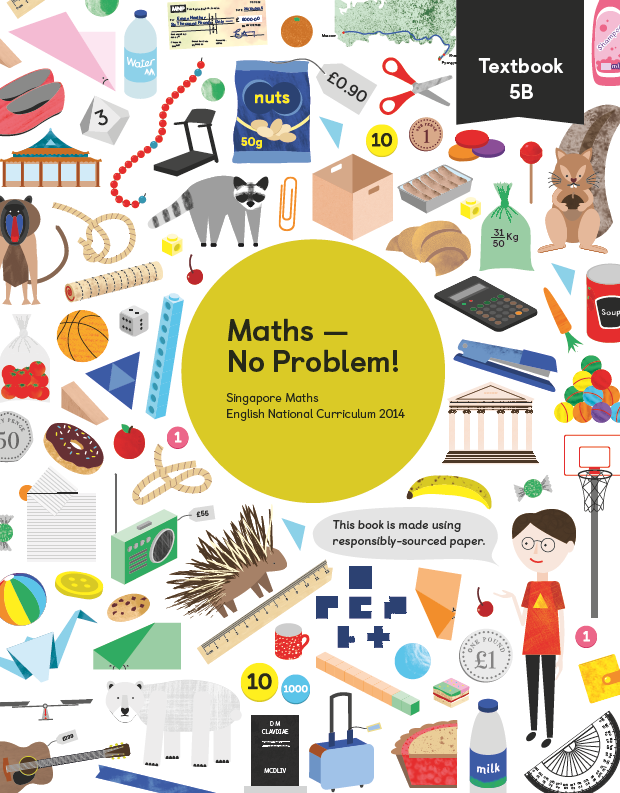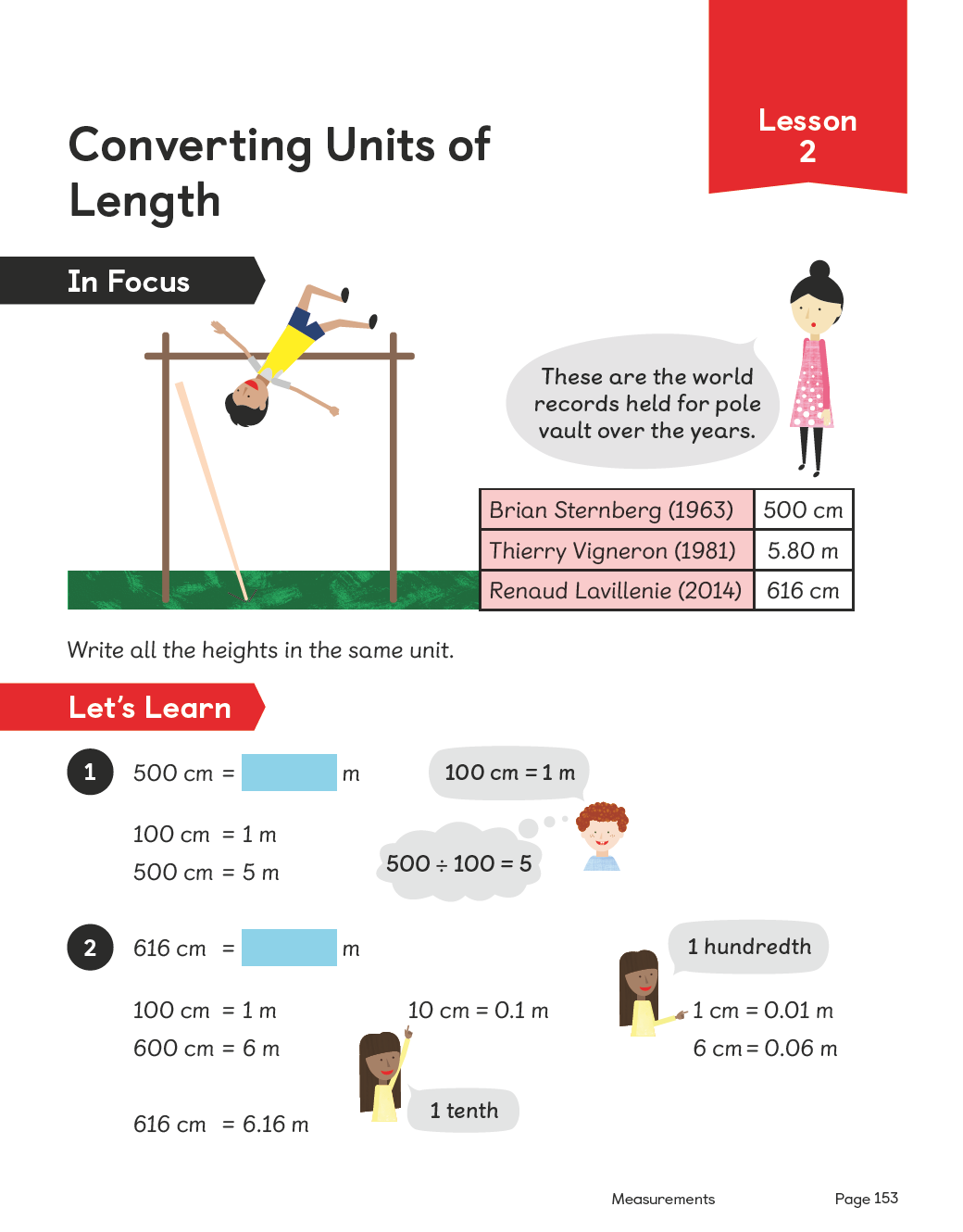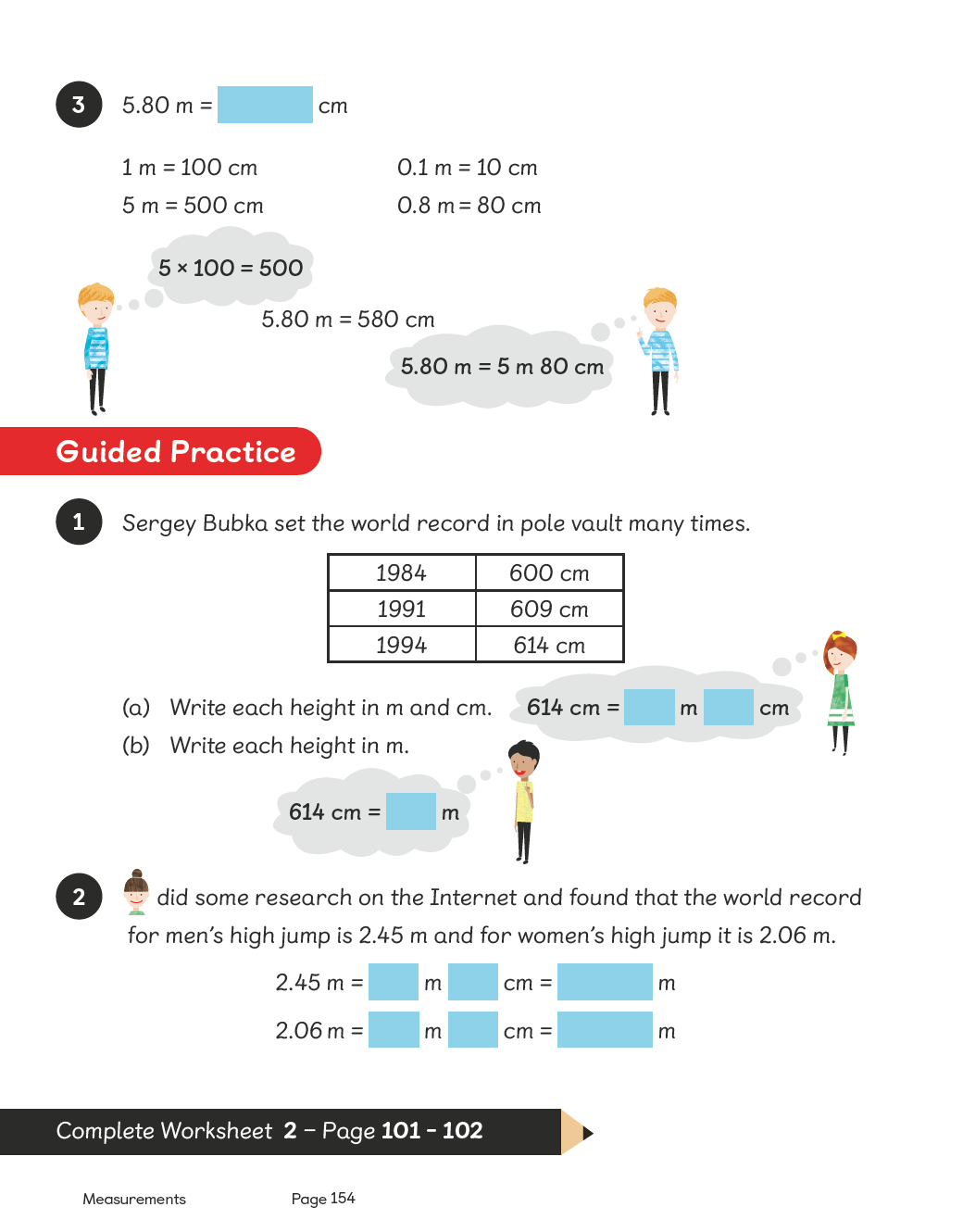Textbook 5B# Maths — No Problem!Textbook 5B

## £9.99

ISBN: 9781910504208
Pages: 274
Availability:
In stock
Qty:

• Lesson 1 - Writing Decimals

To be able to write decimal numbers.

• Lesson 2 - Reading and Writing Decimals

To be able to read and write decimals.

• Lesson 3 - Reading and Writing Decimals

To be able to read and write decimals.

• Lesson 4 - Comparing Decimals

To be able to compare tenths and hundredths written as decimals.

• Lesson 5 - Comparing Decimals

To be able to order and compare decimals.

• Lesson 6 - Comparing Decimals

To be able to compare and order decimals of amounts.

• Lesson 7 - Writing Fractions as Decimals

To be able to write fractions as decimals.

• Lesson 8 - Adding and Subtracting Decimals

To be able to add and subtract amounts in decimals.

• Lesson 9 - Adding and Subtracting Decimals

To be able to add and subtract decimals.
To be able to add and subtract amounts in pounds and pence.

• Lesson 10 - Adding and Subtracting Decimals

To be able to add and subtract amounts in pounds and pence.

• Lesson 11 - Adding and Subtracting Decimals

To be able to add and subtract decimals.
To be able to add and subtract amounts in pounds and pence.

• Lesson 12 - Adding and Subtracting Decimals

To be able to add and subtract decimals to find the smallest possible sum and difference.

• Lesson 13 - Adding and Subtracting Decimals

To be able to add and subtract decimals.
To find number pairs that add up to 1.

• Lesson 14 - Adding and Subtracting Decimals

To be able to add and subtract the perimeter of an object using decimals.

• Lesson 15 - Rounding Decimals

To be able to round decimals to the nearest whole number.
To be able to round numbers to the nearest tenth.

• Lesson 16 - Chapter Consolidation

To be able to apply knowledge of decimals to solve problems.

## Author(s)

Consultant and Author: Dr. Yeap Ban Har
UK Consultant: Dr. Anne Hermanson